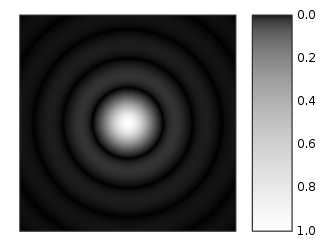•• Carey Smith
• Oxnard College
$$\newcommand{\vecs}{\overset { \rightharpoonup} {\mathbf{#1}} }$$ $$\newcommand{\vecd}{\overset{-\!-\!\rightharpoonup}{\vphantom{a}\smash {#1}}}$$$$\newcommand{\id}{\mathrm{id}}$$ $$\newcommand{\Span}{\mathrm{span}}$$ $$\newcommand{\kernel}{\mathrm{null}\,}$$ $$\newcommand{\range}{\mathrm{range}\,}$$ $$\newcommand{\RealPart}{\mathrm{Re}}$$ $$\newcommand{\ImaginaryPart}{\mathrm{Im}}$$ $$\newcommand{\Argument}{\mathrm{Arg}}$$ $$\newcommand{\norm}{\| #1 \|}$$ $$\newcommand{\inner}{\langle #1, #2 \rangle}$$ $$\newcommand{\Span}{\mathrm{span}}$$ $$\newcommand{\id}{\mathrm{id}}$$ $$\newcommand{\Span}{\mathrm{span}}$$ $$\newcommand{\kernel}{\mathrm{null}\,}$$ $$\newcommand{\range}{\mathrm{range}\,}$$ $$\newcommand{\RealPart}{\mathrm{Re}}$$ $$\newcommand{\ImaginaryPart}{\mathrm{Im}}$$ $$\newcommand{\Argument}{\mathrm{Arg}}$$ $$\newcommand{\norm}{\| #1 \|}$$ $$\newcommand{\inner}{\langle #1, #2 \rangle}$$ $$\newcommand{\Span}{\mathrm{span}}$$$$\newcommand{\AA}{\unicode[.8,0]{x212B}}$$

By Carey A. Smith

There are 2 methods to sum a series. Let the variable "term" be computed value of the current value and the variable "total" be the running sum.

Method 1: Use a temporary variable, "total_new", to make it clear what is happening:

total_new = total + term;  % Add the previous total and the current term to get the new total.

total = total_new;  % Reset total to the new value

Method 2: Directly assign the new value of "total" to be equal to current value of total + term:

total = total + term;  % Replace the previous total with the sum of the current term + term

These 2 methods are equivalent. The first method is more obvious what is happening. The second is method common among experienced programmers. It may be slightly faster.

Note: Variable names other than "total"--such as "series_sum--are often used in codes.

Warning: Do not using "sum" as a variable, because that would be a name collision with the built-in function sum().

.

##### Example $$\PageIndex{1}$$ Power Series1

% Clear any variables etc. clear all; close all; format compact; clc; % Initialize these variables: n = 6;    % The number of terms in the series A0 = 4; % The first value r = 1/2;  % The ratio of successive terms % Write a for loop that computes the terms in the series which are computed with this expression: %   A(k) = A0*r^k;

%% Method 1: total = 0;  % Initialize the total for k = 1:n   A(k) = A0*r^k;   total_new = total + A(k);   total = total_new; end A % This displays all the values of the vector A disp(['Method 1 total = ',num2str(total)])

%% Method 2: total = 0;  % Initialize the total for k = 1:n   A(k) = A0*r^k;   total = total + A(k); end A % This displays all the values of the vector A disp(['Method 2 total = ',num2str(total)])

.

##### Exercise $$\PageIndex{1}$$ atan(x) Taylor's series and absolute error

The Taylor's series for the arctangent function is:

atan(x) = x - x3/3 + x5/5 - x7/7 + ...

The general formula for the kth term is computed with these 2 lines of code:

m = (2*k-1) % This generates 1, 3, 5, 7, ...

term = (-1)^(k-1)*x^m / m   % (-1)^(k-1) = 1, -1, 1, -1, ...

Because the sign changes from term to term, this is called an alternating series.

(1 pt) Write a Matlab m-file script. This script will have a "for loop". The details of the for loop are described below.

(1 pt) Put these lines of code at the beginning of your file:

% Compute the Taylor's series for atan(x) % Clear any variables; close any figures; eliminate white space; clear the console clear all; close all; format compact; clc; % Open a figure for plotting the partial sums

% Octave needs graphics toolkit to plot graph. graphics_toolkit("fltk") % Do not use with MATLAB
figure; hold on; grid on;

(1 pt) Initialize these variables:

x = pi/5 % Set the x-value atan_series = 0; % Initialize the series' sum

(2 pts) Write a for loop. Let k = the for-loop index. k goes from 1 to 8

(3 pts) Inside the for loop, compute term as specified above for each iteration.

Then add term to the previous value of atan_series with this code (method 1):

atan_series_new = atan_series + term

atan_series = atan_series_new

(1 pt) Inside the for loop, also plot the kth partial sum with this code:

plot(k, atan_series,'o');

(1 pt) After the for loop, display the sum and the last term with these lines of code:

atan_series % Display the series sum

term % Display the last term that was calculated

(1 pt) Compute and display atan_Matlab = atan(x) [Matlab's buit-in function]

(1 pt) Compute and display the absolute error = abs( atan_series - atan_Matlab)

If the code is done correctly, the absolute error should be < 0.001

Add texts here. Do not delete this text first

.

##### Example $$\PageIndex{1}$$ Bessel Function

The image of a star thru a space telescope, which has no aberrations, is called an Airy pattern, shown in this image:Figure $$\PageIndex{1}$$: Airy pattern

(https://en.Wikipedia.org/wiki/Airy_disk, This work has been released into the public domain by its author, Sakurambo at English Wikipedia.)

The formula for a cross-section of the Airy pattern intensity is:

$I(\theta) = I_0 \left[ \frac{2J_1(k*a*sin\theta)}{k*a*sin\theta} \right]^2 = I_0 \left[ \frac{2J_1(x)}{x} \right]^2$

where J1(x) is the 1st-order Bessel function of the 1st kind.

This Bessel function is approximated by this infinite series with α = 1:

$J_\alpha (x) = \sum_{m=0}^\infty \frac{(-1)^m}{m!*\Gamma(m+\alpha+1)} * \left(\frac{x}{2}\right)^{(2m+\alpha)}$

For m!, use the Matlab function factorial(m)

Г(p) = the Gamma function. In Matlab this function is gamma(p).

When is a positive integer, gamma(p) = (p-1)!

Instructions:

1. Set x = 0.5
2. Write a for loop to sum the terms of this Bessel function series, Jα(x), for m = 0 to 10.
3. Display the last term
4. Display the resulting sum

Solution

term = 1.5697e-27
J1_x = 0.24227

..

This page titled 3.7.1: Series Additional Material is shared under a CC BY-NC-SA 4.0 license and was authored, remixed, and/or curated by Carey Smith.